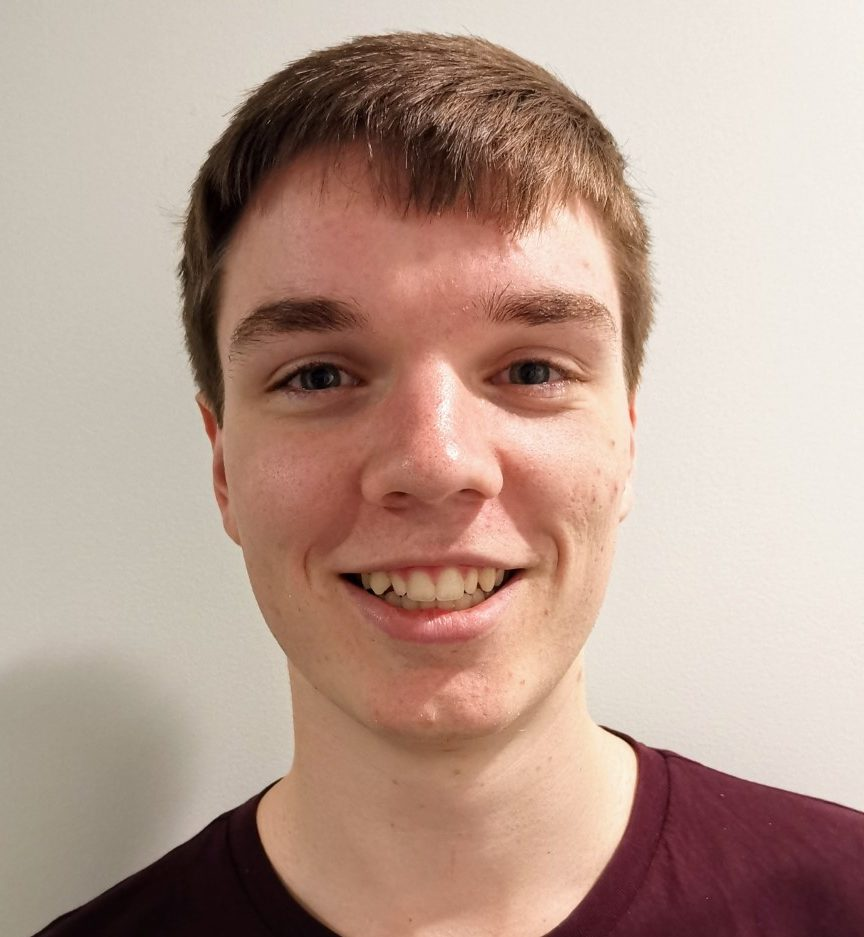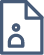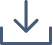### Computational Modelling Using Exponential Integrators

Many physical processes can be modelled using time and space dependent partial differential equations. The solution of these equations provides researchers/industry with valuable insight into the underlying process and can often be used to explain phenomena that has been observed experimentally. However, due to the complexity of these processes and the irregular geometries on which they apply, exact analytical solutions to the governing equations are almost always not accessible. In such cases, numerical solution methods must be called upon to provide an approximate solution. Typically, the first step is to perform a spatial discretisation of the governing equations over the geometry, which produces an initial value problem involving a large system of ordinary differential equations. In recent times, exponential integrators have emerged as an attractive method for solving such initial value problems. In contrast to classical implicit time-stepping schemes (e.g. backward Euler), which require the solution of a nonlinear system of equations at each time step, exponential integrators are explicit schemes that require the computation of matrix functions involving the matrix exponential. This project will involve developing efficient exponential integration schemes, and related numerical linear algebra techniques, for solving several classical partial differential equations that arise frequently in applied mathematics.#### Joshua Wilson

Queensland University of Technology

Joshua Wilson is a third-year undergraduate student studying at Queensland University of Technology. Undertaking a Bachelor of Engineering / Mathematics, he is interested in how computation and simulation can be applied to great effect across a variety of disciplines. Similarly, he has a keen interest in pursuing further studies after he has finished his undergraduate degree, having previously completed a QUT VRES project into cryptography. In his spare time, Joshua enjoys solving problems, playing games, and generally having a good time with friends.